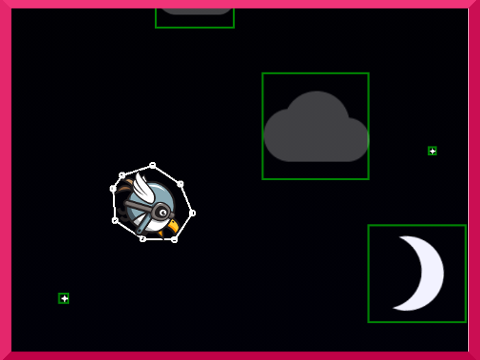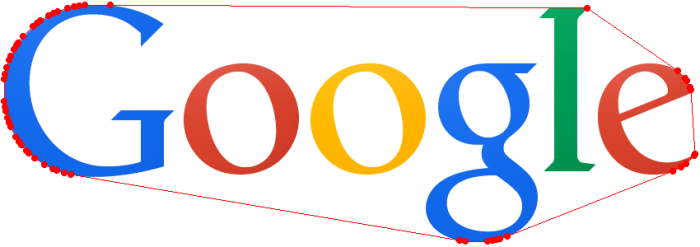# Android, Bitmaps, and Convex HullIn a game I’m currently working on (Lollipop Land) I set out to add more unique characters. The object of the game is to jump/fly your way through lollipops without hitting one or face planting onto the ground.

The next release will have at least 11 new characters. Each character is a set of PNGs that make up an AnimatedDrawable. The new characters are different shapes and sizes, thus adding complexity to when the character actually intersects with an obstacle in the game. After some research, I came across the convex hull algorithm. Ultimately, my goal was to eliminate any transparency in the image for the best precision when passing through obstacles in the game. The convex hull algorithm is exactly what I was looking for.

## What Is The Convex Hull Algorithm?

If you had an image or object and put a rubber band around that object, the convex hull would be the set of points at which the rubber band touched the object. Here is a more thorough definition:

In mathematics, the convex hull or convex envelope of a set X of points in the Euclidean plane or Euclidean space is the smallest convex set that contains X. For instance, when X is a bounded subset of the plane, the convex hull may be visualized as the shape formed by a rubber band stretched around X.

Formally, the convex hull may be defined as the intersection of all convex sets containing X or as the set of all convex combinations of points in X. With the latter definition, convex hulls may be extended from Euclidean spaces to arbitrary real vector spaces; they may also be generalized further, to oriented matroids.1, 2

## Finding X and Y Boundaries for a Bitmap

First off, you need to load your bitmap or drawable into memory. Once we have a reference to the bitmap we can parse the bitmap pixel by pixel.3

#### Find the non-transparent coordinates:

ArrayList<Point> points = new ArrayList<Point>();
int width = bitmap.getWidth();
int height = bitmap.getHeight();
int[] pixels = new int[width * height];
bitmap.getPixels(pixels, 0, width, 0, 0, width, height);
for (int x = 0; x < width; x++) {
int firstY = -1, lastY = -1;
for (int y = 0; y < height; y++) {
boolean transparent = (pixels[y * width + x] == Color.TRANSPARENT);
if (!transparent) {
if (firstY == -1) {
firstY = y;
}
lastY = y;
}
}
if (firstY != -1) {
}
}


## QuickHull

The code above will give us a path around our bitmap, but the set of points will be much larger than desired. To increase performance we can use the Quickhull algorithm. Quickhull is a method of computing the convex hull of a finite set of points in n-dimensional space. It uses a divide and conquer approach similar to that of quicksort, from which its name derives. Its worst case complexity for 2-dimensional and 3-dimensional space is considered to be $$O(n\log(r))$$, where n is the number of input points and r is the number of processed points.4

Here is my modified Quickhull class for Android:

### Implementation

##### QuickHull.java (Licensed under Apache 2.0)
/*
* Copyright (C) 2014 Jared Rummler <[email protected]>
*
* you may not use this file except in compliance with the License.
* You may obtain a copy of the License at
*
*
* Unless required by applicable law or agreed to in writing, software
* WITHOUT WARRANTIES OR CONDITIONS OF ANY KIND, either express or implied.
* See the License for the specific language governing permissions and
*/

import java.util.ArrayList;

import android.graphics.Bitmap;
import android.graphics.Bitmap.Config;
import android.graphics.Canvas;
import android.graphics.Color;
import android.graphics.Point;
import android.graphics.drawable.BitmapDrawable;
import android.graphics.drawable.Drawable;
import android.support.annotation.NonNull;

/**
* Find points in convex hull using quick hull method
*/
public class QuickHull {

/** Returns the quick hull of a {@link Drawable}, which is scaled down by the size */
public static ArrayList<Point> quickHull(@NonNull Drawable drawable, int size) {
return quickHull(Bitmap.createScaledBitmap(drawableToBitmap(drawable), size, size, false));
}

/** Returns the quick hull of a {@link Drawable} */
public static ArrayList<Point> quickHull(@NonNull Drawable drawable) {
final ArrayList<Point> hull = new ArrayList<Point>();
final Bitmap bitmap = drawableToBitmap(drawable);
if (bitmap != null) {
}
return hull;
}

/** Returns the quick hull of a bitmap */
public static ArrayList<Point> quickHull(@NonNull Bitmap bitmap) {
final ArrayList<Point> points = new ArrayList<Point>();
final int width = bitmap.getWidth();
final int height = bitmap.getHeight();
final int[] pixels = new int[width * height];
bitmap.getPixels(pixels, 0, width, 0, 0, width, height);
for (int x = 0; x < width; x++) {
int firstY = -1, lastY = -1;
for (int y = 0; y < height; y++) {
final boolean transparent = (pixels[y * width + x] == Color.TRANSPARENT);
if (!transparent) {
if (firstY == -1) {
firstY = y;
}
lastY = y;
}
}
if (firstY != -1) {
}
}
return quickHull(points);
}

/** Returns a list of points in convex hull using quick hull method */
@SuppressWarnings("unchecked")
public static ArrayList<Point> quickHull(ArrayList<Point> points) {
final ArrayList<Point> convexHull = new ArrayList<Point>();
if (points.size() < 3) {
return (ArrayList<Point>) points.clone();
}

int minPoint = -1, maxPoint = -1;
int minX = Integer.MAX_VALUE;
int maxX = Integer.MIN_VALUE;
for (int i = 0; i < points.size(); i++) {
if (points.get(i).x < minX) {
minX = points.get(i).x;
minPoint = i;
}
if (points.get(i).x > maxX) {
maxX = points.get(i).x;
maxPoint = i;
}
}
final Point a = points.get(minPoint);
final Point b = points.get(maxPoint);
points.remove(a);
points.remove(b);

ArrayList<Point> leftSet = new ArrayList<Point>();
ArrayList<Point> rightSet = new ArrayList<Point>();

for (int i = 0; i < points.size(); i++) {
Point p = points.get(i);
if (pointLocation(a, b, p) == -1) leftSet.add(p);
}
hullSet(a, b, rightSet, convexHull);
hullSet(b, a, leftSet, convexHull);

return convexHull;
}

private static int distance(Point a, Point b, Point c) {
final int ABx = b.x - a.x;
final int ABy = b.y - a.y;
int num = ABx * (a.y - c.y) - ABy * (a.x - c.x);
if (num < 0) num = -num;
return num;
}

private static void hullSet(Point a, Point b, ArrayList<Point> set, ArrayList<Point> hull) {
final int insertPosition = hull.indexOf(b);
if (set.size() == 0) return;
if (set.size() == 1) {
final Point p = set.get(0);
set.remove(p);
return;
}
int dist = Integer.MIN_VALUE;
int furthestPoint = -1;
for (int i = 0; i < set.size(); i++) {
Point p = set.get(i);
int distance = distance(a, b, p);
if (distance > dist) {
dist = distance;
furthestPoint = i;
}
}
final Point p = set.get(furthestPoint);
set.remove(furthestPoint);

// Determine who's to the left of AP
final ArrayList<Point> leftSetAP = new ArrayList<Point>();
for (int i = 0; i < set.size(); i++) {
final Point m = set.get(i);
if (pointLocation(a, p, m) == 1) {
}
}

// Determine who's to the left of PB
final ArrayList<Point> leftSetPB = new ArrayList<Point>();
for (int i = 0; i < set.size(); i++) {
final Point m = set.get(i);
if (pointLocation(p, b, m) == 1) {
}
}
hullSet(a, p, leftSetAP, hull);
hullSet(p, b, leftSetPB, hull);
}

private static int pointLocation(Point a, Point b, Point p) {
int cp1 = (b.x - a.x) * (p.y - a.y) - (b.y - a.y) * (p.x - a.x);
return (cp1 > 0) ? 1 : -1;
}

private static Bitmap drawableToBitmap(@NonNull Drawable drawable) {
if (drawable instanceof BitmapDrawable) {
return ((BitmapDrawable) drawable).getBitmap();
}
final int height = drawable.getIntrinsicHeight();
final int width = drawable.getIntrinsicWidth();
if (width <= 0 || height <= 0) {
return null;
}
final Bitmap bitmap = Bitmap.createBitmap(width, height, Config.ARGB_8888);
final Canvas canvas = new Canvas(bitmap);
drawable.setBounds(0, 0, canvas.getWidth(), canvas.getHeight());
drawable.draw(canvas);
return bitmap;
}

private QuickHull() {
throw new IllegalStateException("no instances");
}

}


### QuickHull Example Usage:

Now with this helper class we can get the convex hull of a Bitmap on Android with a single line of code.

List<Point> hull = QuickHull.quickHull(bitmap);


Let’s test out the Google logo to see the “rubber band” in action.